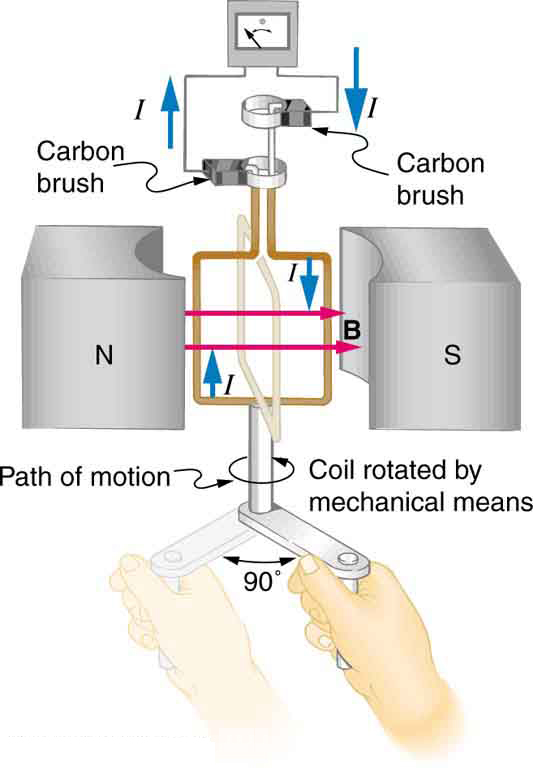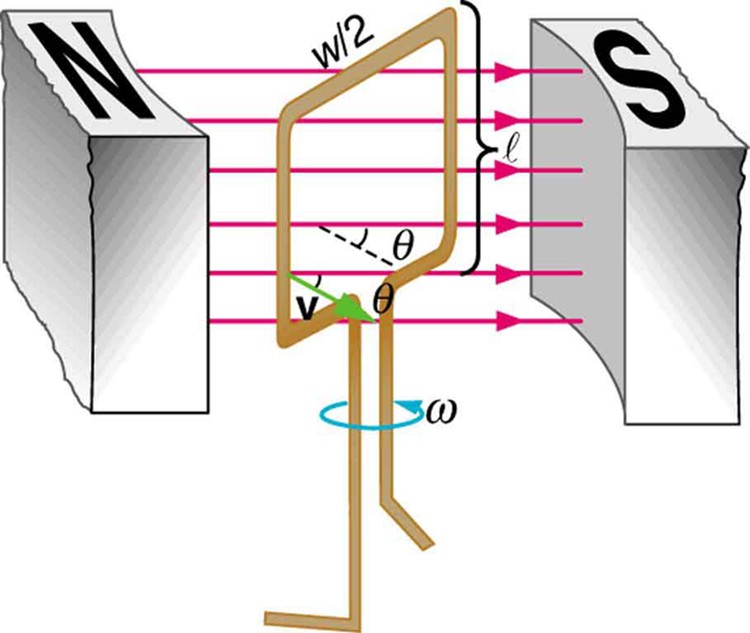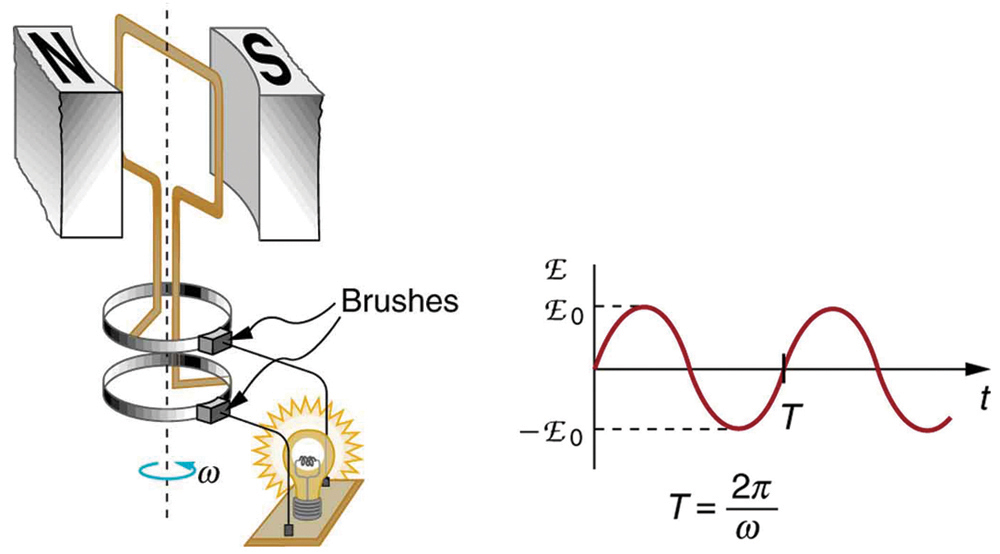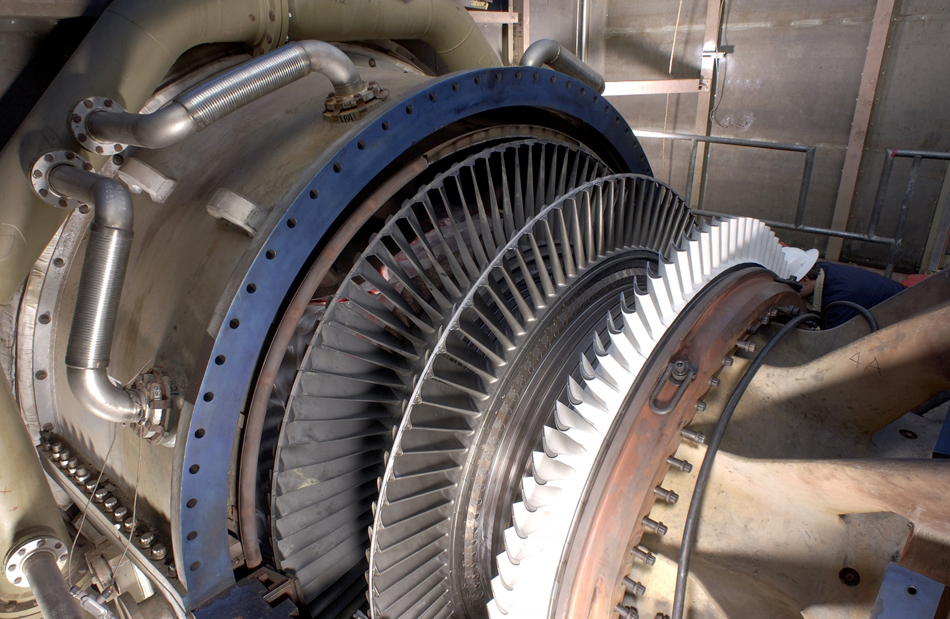Chapter 23 Electromagnetic Induction, AC Circuits, and Electrical Technologies

# 23.5 Electric Generators

Electric Generators
• Calculate the emf induced in a generator.
• Calculate the peak emf which can be induced in a particular generator system.

Electric generators induce an emf by rotating a coil in a magnetic field, as briefly discussed in Induced Emf and Magnetic Flux. We will now explore generators in more detail. Consider the following example.

Calculating the Emf Induced in a Generator Coil

The generator coil shown in [link] is rotated through one-fourth of a revolution (from θ=0ºθ=0º
to θ=90ºθ=90º
) in 15.0 ms. The 200-turn circular coil has a 5.00 cm radius and is in a uniform 1.25 T magnetic field. What is the average emf induced?When this generator coil is rotated through one-fourth of a revolution, the magnetic flux ΦΦ changes from its maximum to zero, inducing an emf.

Strategy

We use Faraday’s law of induction to find the average emf induced over a time ΔtΔt size 12{Δt} {}:

emf=−NΔΦΔt.emf=−NΔΦΔt. size 12{“emf”= – N { {ΔΦ} over {Δt} } } {}

We know that N=200N=200 size 12{N=”200″} {} and Δt=15.0msΔt=15.0ms size 12{Δt=”15″ “.” 0`”ms”} {}, and so we must determine the change in flux ΔΦΔΦ size 12{ΔΦ} {} to find emf.

Solution

Since the area of the loop and the magnetic field strength are constant, we see that

ΔΦ=Δ(BAcosθ)=ABΔ(cosθ).ΔΦ=Δ(BAcosθ)=ABΔ(cosθ). size 12{ΔΦ=Δ ( ital “BA””cos”θ ) = ital “AB”Δ ( “cos”θ ) } {}

Now, Δ(cosθ)=−1.0Δ(cosθ)=−1.0 size 12{Δ ( “cos”θ ) = – 1 “.” 0} {}, since it was given that θθ goes from 0º0º to 90º90º . Thus ΔΦ=−ABΔΦ=−AB size 12{ΔΦ= – ital “AB”} {}, and

emf=NABΔt.emf=NABΔt. size 12{“emf”=N { { ital “AB”} over {Δt} } } {}

The area of the loop is A=πr2=(3.14…)(0.0500m)2=7.85×10−3m2A=πr2=(3.14…)(0.0500m)2=7.85×10−3m2 size 12{A=πr rSup { size 8{2} } = ( 3 “.” “14” “.” “.” “.” ) ( 0 “.” “0500”`m ) rSup { size 8{2} } =7 “.” “85” times “10” rSup { size 8{ – 3} } `m rSup { size 8{2} } } {}. Entering this value gives

emf=200(7.85×10−3m2)(1.25 T)15.0×10−3 s=131V.emf=200(7.85×10−3m2)(1.25 T)15.0×10−3 s=131V. size 12{“emf”=”200” { { ( 7 “.” “85” times “10” rSup { size 8{ – 3} } ” m” rSup { size 8{2} } ) ( 1 “.” “25”” T” ) } over {“15” “.” 0 times “10” rSup { size 8{ – 3} } ” s”} } =”131″” V”} {}

Discussion

This is a practical average value, similar to the 120 V used in household power.

The emf calculated in [link] is the average over one-fourth of a revolution. What is the emf at any given instant? It varies with the angle between the magnetic field and a perpendicular to the coil. We can get an expression for emf as a function of time by considering the motional emf on a rotating rectangular coil of width ww size 12{w} {} and height ℓℓ size 12{l} {} in a uniform magnetic field, as illustrated in [link].A generator with a single rectangular coil rotated at constant angular velocity in a uniform magnetic field produces an emf that varies sinusoidally in time. Note the generator is similar to a motor, except the shaft is rotated to produce a current rather than the other way around.

Charges in the wires of the loop experience the magnetic force, because they are moving in a magnetic field. Charges in the vertical wires experience forces parallel to the wire, causing currents. But those in the top and bottom segments feel a force perpendicular to the wire, which does not cause a current. We can thus find the induced emf by considering only the side wires. Motional emf is given to be emf=Bℓvemf=Bℓv size 12{“emf”=Bℓv} {}, where the velocity v is perpendicular to the magnetic field BB size 12{B} {}. Here the velocity is at an angle θθ size 12{θ} {} with BB size 12{B} {}, so that its component perpendicular to BB size 12{B} {} is vsinθvsinθ size 12{v”sin”θ} {} (see [link]). Thus in this case the emf induced on each side is emf=Bℓvsinθemf=Bℓvsinθ size 12{“emf”=Bℓv”sin”θ} {}, and they are in the same direction. The total emf around the loop is then

emf=2Bℓvsinθ.emf=2Bℓvsinθ. size 12{“emf”=2Bℓv”sin”θ} {}

This expression is valid, but it does not give emf as a function of time. To find the time dependence of emf, we assume the coil rotates at a constant angular velocity ωω size 12{ω} {}. The angle θθ size 12{θ} {} is related to angular velocity by θ=ωtθ=ωt size 12{θ=ωt} {}, so that

emf=2Bℓvsinωt.emf=2Bℓvsinωt. size 12{“emf”=Bℓv”sin”ωt} {}

Now, linear velocity
vv
is related to angular velocity
ωω by v=rωv=rω size 12{v=rω} {}. Here r=w/2r=w/2 size 12{r=w/2} {}, so that v=(w/2)ωv=(w/2)ω size 12{v= ( w/2 ) ω} {}, and

emf=2Bℓw2ωsinωt=(ℓw)Bωsinωt.emf=2Bℓw2ωsinωt=(ℓw)Bωsinωt. size 12{“emf”=2Bℓ { {w} over {2} } ω”sin”ωt= ( ℓw ) Bω”sin”ωt} {}

Noting that the area of the loop is A=ℓwA=ℓw size 12{A=ℓw} {}, and allowing for NN size 12{N} {} loops, we find that

emf=NABωsinωtemf=NABωsinωt size 12{“emf”= ital “NAB”ω”sin”ωt} {}

is the emf induced in a generator coil of NN size 12{N} {} turns and area AA size 12{A} {} rotating at a constant angular velocity
ωω
in a uniform magnetic field BB size 12{B} {}. This can also be expressed as

emf=emf0sinωt,emf=emf0sinωt, size 12{“emf”=”emf” rSub { size 8{0} } “sin”ωt} {}

where

emf0=NABωemf0=NABω size 12{“emf” rSub { size 8{0} } = ital “NAB”ω} {}

is the maximum (peak) emf. Note that the frequency of the oscillation is f=ω/2πf=ω/2π size 12{f=ω/2π} {}, and the period is T=1/f=2π/ωT=1/f=2π/ω size 12{T=1/f=2π/ω} {}. [link] shows a graph of emf as a function of time, and it now seems reasonable that AC voltage is sinusoidal.The emf of a generator is sent to a light bulb with the system of rings and brushes shown. The graph gives the emf of the generator as a function of time. emf0emf0 size 12{“emf” rSub { size 8{0} } } {} is the peak emf. The period is T=1/f=2π/ωT=1/f=2π/ω size 12{T=1/f=2π/ω} {}, where ff size 12{f} {} is the frequency. Note that the script E stands for emf.

The fact that the peak emf, emf0=NABωemf0=NABω size 12{“emf” rSub { size 8{0} } = ital “NAB”ω} {}, makes good sense. The greater the number of coils, the larger their area, and the stronger the field, the greater the output voltage. It is interesting that the faster the generator is spun (greater ωω size 12{ω} {}), the greater the emf. This is noticeable on bicycle generators—at least the cheaper varieties. One of the authors as a juvenile found it amusing to ride his bicycle fast enough to burn out his lights, until he had to ride home lightless one dark night.

[link] shows a scheme by which a generator can be made to produce pulsed DC. More elaborate arrangements of multiple coils and split rings can produce smoother DC, although electronic rather than mechanical means are usually used to make ripple-free DC.

Calculating the Maximum Emf of a Generator

Calculate the maximum emf, emf0emf0 size 12{“emf” rSub { size 8{0} } } {}, of the generator that was the subject of [link].

Strategy

Once ωω size 12{ω} {}, the angular velocity, is determined, emf0=NABωemf0=NABω size 12{“emf” rSub { size 8{0} } = ital “NAB”ω} {} can be used to find emf0emf0 size 12{“emf” rSub { size 8{0} } } {}. All other quantities are known.

Solution

Angular velocity is defined to be the change in angle per unit time:

ω=ΔθΔt.ω=ΔθΔt. size 12{ω= { {Δθ} over {Δt} } } {}

One-fourth of a revolution is π/2π/2 size 12{l} {} radians, and the time is 0.0150 s; thus,

104.7 rad/s is exactly 1000 rpm. We substitute this value for ωω size 12{ω} {} and the information from the previous example into emf0=NABωemf0=NABω size 12{“emf” rSub { size 8{0} } = ital “NAB”ω} {}, yielding

size 12{“emf” rSub { size 8{0} } = ital “NAB”ω} {} #
” “=”200” ( 7 “.” “85” times “10” rSup { size 8{ – 3} } ” m” rSup { size 8{2} } ) ( 1 “.” “25”” T” ) ( “104” “.” 7″ rad/s” ) {} #
” “=”206″” V” {}
} } {}

Discussion

The maximum emf is greater than the average emf of 131 V found in the previous example, as it should be.

In real life, electric generators look a lot different than the figures in this section, but the principles are the same. The source of mechanical energy that turns the coil can be falling water (hydropower), steam produced by the burning of fossil fuels, or the kinetic energy of wind. [link] shows a cutaway view of a steam turbine; steam moves over the blades connected to the shaft, which rotates the coil within the generator.Steam turbine/generator. The steam produced by burning coal impacts the turbine blades, turning the shaft which is connected to the generator. (credit: Nabonaco, Wikimedia Commons)

Generators illustrated in this section look very much like the motors illustrated previously. This is not coincidental. In fact, a motor becomes a generator when its shaft rotates. Certain early automobiles used their starter motor as a generator. In Back Emf, we shall further explore the action of a motor as a generator.

# Section Summary

• An electric generator rotates a coil in a magnetic field, inducing an emfgiven as a function of time by
emf=NABωsinωt,emf=NABωsinωt, size 12{“emf”= ital “NAB”ω”sin”ωt} {}
where AA size 12{A} {} is the area of an NN size 12{N} {}-turn coil rotated at a constant angular velocity ωω size 12{ω} {} in a uniform magnetic field BB size 12{B} {}.
• The peak emf emf0emf0 size 12{“emf” rSub { size 8{0} } } {} of a generator is
emf0=NABω.emf0=NABω. size 12{“emf” rSub { size 8{0} } = ital “NAB”ω} {}

# Conceptual Questions

Using RHR-1, show that the emfs in the sides of the generator loop in [link] are in the same sense and thus add.

The source of a generator’s electrical energy output is the work done to turn its coils. How is the work needed to turn the generator related to Lenz’s law?

# Problems & Exercises

Calculate the peak voltage of a generator that rotates its 200-turn, 0.100 m diameter coil at 3600 rpm in a 0.800 T field.

474 V

At what angular velocity in rpm will the peak voltage of a generator be 480 V, if its 500-turn, 8.00 cm diameter coil rotates in a 0.250 T field?

What is the peak emf generated by rotating a 1000-turn, 20.0 cm diameter coil in the Earth’s 5.00×10−5T5.00×10−5T size 12{5 “.” “00” times “10” rSup { size 8{ – 5} } `T} {} magnetic field, given the plane of the coil is originally perpendicular to the Earth’s field and is rotated to be parallel to the field in 10.0 ms?

0.247 V

What is the peak emf generated by a 0.250 m radius, 500-turn coil is rotated one-fourth of a revolution in 4.17 ms, originally having its plane perpendicular to a uniform magnetic field. (This is 60 rev/s.)

(a) A bicycle generator rotates at 1875 rad/s, producing an 18.0 V peak emf. It has a 1.00 by 3.00 cm rectangular coil in a 0.640 T field. How many turns are in the coil? (b) Is this number of turns of wire practical for a 1.00 by 3.00 cm coil?

(a) 50

(b) yes

Integrated Concepts

This problem refers to the bicycle generator considered in the previous problem. It is driven by a 1.60 cm diameter wheel that rolls on the outside rim of the bicycle tire. (a) What is the velocity of the bicycle if the generator’s angular velocity is 1875 rad/s? (b) What is the maximum emf of the generator when the bicycle moves at 10.0 m/s, noting that it was 18.0 V under the original conditions? (c) If the sophisticated generator can vary its own magnetic field, what field strength will it need at 5.00 m/s to produce a 9.00 V maximum emf?

(a) A car generator turns at 400 rpm when the engine is idling. Its 300-turn, 5.00 by 8.00 cm rectangular coil rotates in an adjustable magnetic field so that it can produce sufficient voltage even at low rpms. What is the field strength needed to produce a 24.0 V peak emf? (b) Discuss how this required field strength compares to those available in permanent and electromagnets.

(a) 0.477 T

(b) This field strength is small enough that it can be obtained using either a permanent magnet or an electromagnet.

Show that if a coil rotates at an angular velocity ωω, the period of its AC output is
2π/ω2π/ω.

A 75-turn, 10.0 cm diameter coil rotates at an angular velocity of 8.00 rad/s in a 1.25 T field, starting with the plane of the coil parallel to the field. (a) What is the peak emf? (b) At what time is the peak emf first reached? (c) At what time is the emf first at its most negative? (d) What is the period of the AC voltage output?

(a) 5.89 V

(b) At t=0

(c) 0.393 s

(d) 0.785 s

(a) If the emf of a coil rotating in a magnetic field is zero at t=0t=0 size 12{t=0} {}, and increases to its first peak at t=0.100mst=0.100ms size 12{t=0 “.” “100”`”ms”} {}, what is the angular velocity of the coil? (b) At what time will its next maximum occur? (c) What is the period of the output? (d) When is the output first one-fourth of its maximum? (e) When is it next one-fourth of its maximum?

Unreasonable Results

A 500-turn coil with a 0.250m20.250m2 size 12{0 “.” “250”`m rSup { size 8{2} } } {} area is spun in the Earth’s 5.00×10−5T5.00×10−5T size 12{5 “.” “00” times “10” rSup { size 8{ – 5} } `T} {} field, producing a 12.0 kV maximum emf. (a) At what angular velocity must the coil be spun? (b) What is unreasonable about this result? (c) Which assumption or premise is responsible?

(b) This angular velocity is unreasonably high, higher than can be obtained for any mechanical system.

(c) The assumption that a voltage as great as 12.0 kV could be obtained is unreasonable.

## Glossary

electric generator
a device for converting mechanical work into electric energy; it induces an emf by rotating a coil in a magnetic field
emf induced in a generator coil
emf=NABωsinωtemf=NABωsinωt size 12{“emf”= ital “NAB”ω”sin”ωt} {}, where AA is the area of an NN-turn coil rotated at a constant angular velocity
ωω in a uniform magnetic field BB, over a period of time tt
peak emf
emf0=NABωemf0=NABω size 12{“emf” rSub { size 8{0} } = ital “NAB”ω} {}